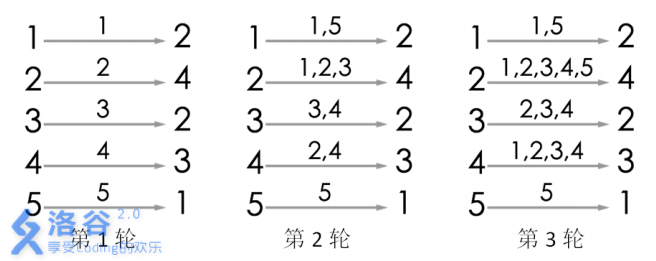# P2661 信息传递

• 15.1K通过
• 45K提交
• 题目提供者 CCF_NOI
• 评测方式 云端评测
• 标签 图论 并查集 NOIp提高组 2015
• 难度 普及/提高-
• 时空限制 1000ms / 128MB
• 提示：收藏到任务计划后，可在首页查看。

## 题目描述

有 $n$ 个同学（编号为 $1$ 到 $n$ ）正在玩一个信息传递的游戏。在游戏里每人都有一个固定的信息传递对象，其中，编号为 $i$ 的同学的信息传递对象是编号为 $T_i$ 的同学。

游戏开始时，每人都只知道自己的生日。之后每一轮中，所有人会同时将自己当前所知的生日信息告诉各自的信息传递对象（注意：可能有人可以从若干人那里获取信息， 但是每人只会把信息告诉一个人，即自己的信息传递对象）。当有人从别人口中得知自 己的生日时，游戏结束。请问该游戏一共可以进行几轮？

## 输入输出格式

输入格式：

共$2$行。

第$1$行包含1个正整数 $n$ ，表示 $n$ 个人。

第$2$行包含 $n$ 个用空格隔开的正整数 $T_1,T_2,\cdots\cdots,T_n$ ，其中第 $i$ 个整数 $T_i$ 表示编号为 $i$ 的同学的信息传递对象是编号为 $T_i$ 的同学， $T_i \leq n$ 且 $T_i \neq i$ 。

输出格式：

$1$个整数，表示游戏一共可以进行多少轮。

## 输入输出样例

输入样例#1： 复制
5
2 4 2 3 1
输出样例#1： 复制
3

## 说明

样例1解释游戏的流程如图所示。当进行完第$3$ 轮游戏后， $4$号玩家会听到 $2$ 号玩家告诉他自己的生日，所以答案为 $3$。当然，第 $3$ 轮游戏后，$2$号玩家、 $3$ 号玩家都能从自己的消息来源得知自己的生日，同样符合游戏结束的条件。

对于 $30\%$的数据， $n ≤ 200$；

对于 $60\%$的数据， $n ≤ 2500$；

对于$100\%$的数据， $n ≤ 200000$。

提示
标程仅供做题后或实在无思路时参考。
请自觉、自律地使用该功能并请对自己的学习负责。
如果发现恶意抄袭标程，将按照I类违反进行处理。